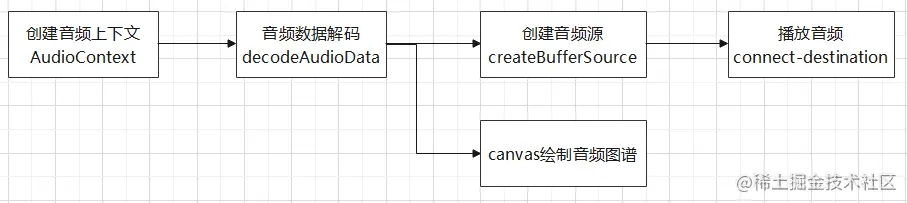﻿ 一文详解Web Audi 绘制音频图谱_javascript技巧_脚本之家
javascript技巧# 一文详解Web Audi 绘制音频图谱

## 实现思路

### 获取音频文件

```// 音频url
let audioUrl = 'https://wavesurfer-js.org/example/media/demo.wav';
// 创建音频上下文
let audioCtx = new (window.AudioContext || window.webkitAudioContext)();
// 创建音频源
let source = audioCtx.createBufferSource();
/*
* 通过fetch下载音频，responseType设置为'arrayBuffer'，我们以arrayBuffer格式接收返回的数据
*/
fetch(audioUrl, {
method: 'GET',
responseType: 'arraybuffer',
}).then(res => {
return res.arrayBuffer();
}).then(data => {
// 处理音频数据
initAudio(data);
});
```

### 利用Web Audio Api 处理音频数据```// audio 初始化
function initAudio (data) {
// 音频数据解码
// decodeAudioData方法接收一个arrayBuffer数据作为参数，这也是为什么前面fetch音频时设置以arrayBuffer格式接收数据
audioCtx.decodeAudioData(data).then(buffer => {
// decodeAudioData解码完成后，返回一个AudioBuffer对象
// 绘制音频波形图
drawWave(buffer);
// 连接音频源
source.buffer = buffer;
source.connect(audioCtx.destination);
// 音频数据处理完毕
});
}
// web audio 规范不允许音频自动播放，需要用户触发页面事件来触发播放，这里我们增加一个播放按钮，数据处理完毕后点击播放
document.querySelector('#btn').onclick = () => {
// 播放音频
source.start(0);
}
```

### 通过解码后的音频数据，绘制波形图

```// 绘制波形图
function drawWave (buffer) {
// buffer.numberOfChannels返回音频的通道数量，1即为单声道，2代表双声道。这里我们只取一条通道的数据
let data = [];
let originData = buffer.getChannelData(0);
// 存储所有的正数据
let positives = [];
// 存储所有的负数据
let negatives = [];
// 先每隔100条数据取1条
for (let i = 0; i < originData.length; i += 100) {
data.push(originData[i]);
}
// 再从data中每10条取一个最大值一个最小值
for (let j = 0, len = parseInt(data.length / 10); j < len; j++) {
let temp = data.slice(j * 10, (j + 1) * 10);
positives.push(Math.max.apply(null, temp));
negatives.push(Math.min.apply(null, temp));
}
// 创建canvas上下文
let canvas = document.querySelector('#canvas');
if (canvas.getContext) {
let ctx = canvas.getContext('2d');
canvas.width = positives.length;
let x = 0;
let y = 100;
let offset = 0;
ctx.fillStyle = '#fa541c';
ctx.beginPath();
ctx.moveTo(x, y);
// canvas高度200，横坐标在canvas中点100px的位置，横坐标上方绘制正数据，下方绘制负数据
// 先从左往右绘制正数据
// x + 0.5是为了解决canvas 1像素线条模糊的问题
for (let k = 0; k < positives.length; k++) {
ctx.lineTo(x + k + 0.5, y - (100 * positives[k]));
}
// 再从右往左绘制负数据
for (let l = negatives.length - 1; l >= 0; l--) {
ctx.lineTo(x + l + 0.5, y + (100 * Math.abs(negatives[l])));
}
// 填充图形
ctx.fill();
}
};
```